# SSC CGL Prelims Quantitative Aptitude Question Paper 2021

• Last Updated : 02 Jul, 2022

SSC CGL provides government jobs in group B and group C posts in ministries and departments of the Government of India. SSC CGL recruitment procedure is completed in three phases – MCQ Based (Tier I and Tier II), Description (Hindi/English), and Computer proficiency test/skill test. To get proper practice and ideas for questions of CGL Tier I, you must attempt the previous year’s question papers. Here We provide the Tier I Quant Section question paper of 2021.

Que.1 The average weight of a boy and his three friends is 55 kg. If the boy is 4 kg more than the average weight of his three friends, what is the weight of the boy ( in kg)?

A) 54 kg

B) 60 kg

C) 58 kg

D) 62 kg

Explanation:

Total weight of the boy and his three friends =55× 4 = 220 kg

Let, the average weight of three friends = x kg

Total weight of three friends = 3x kg

The boy’s weight = ( x + 4) kg

According to question,

(x + 4) + 3x = 220

=> x = 216/4 = 54 kg

∴ The weight of the boy = (54 + 4) = 58 kg.

Que.2 The angle of elevation of the top of an unfinished tower at a point distant 78 m from its base is 30°. How much higher must the tower be raised ( in m) so that the angle of elevation of the top of the finished tower at the same point will be 60°?

A) 52√3

B) 80

C) 26√3

D) 78√3

Explanation: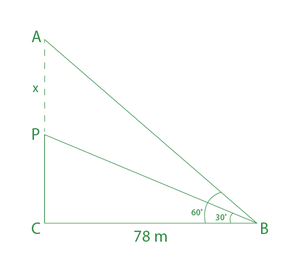Here in fig, PC = Unfinished tower

Now, from ∆PBC,

tan30° = PC/BC = PC/78

=> 1/√3 = PC/78

=> PC = 78/√3 = 78√3/(√3×√3)  (multiply by √3)

∴PC = 26√3

Let, the height of the tower will be raised by x.

So AP = x

Now, ∆ABC

tan60° = AC/BC = (AP+PC)/78

=> √3 = (AP+PC)/78

=> AP = 78√3 – 26√3  ( putting the value of PC)

∴ AP = 52√3  m

∴ The height of the tower must be raised by 52√3 m.

Shortcut method :-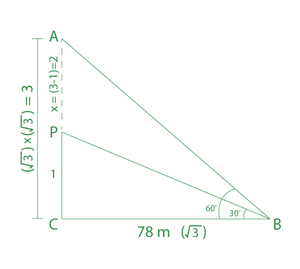tan30° = 1/√3 = PC/BC

It means PC = 1 unit & BC = √3 unit

tan60° = √3 = AC/BC

AC = √3 unit and BC = 1 unit

But BC = √3 unit, so AC = √3×√3 = 3 unit

AC = AP + PC

=> 3 = AP + 1

=> AP = 2 unit

Now, according to question,

√3 = 78

=> 2 unit = 78/√3 × 2 m = 52√3 m

∴ The height of the tower must be raised by 52√3 m.

Que.3 A sold a mobile phone to B at a gain of 25% and B sold it to C at a loss of 10%. If C paid Rs.5625 for it, how much did A pay (in Rs) for the phone?

A) 5000

B) 4500

C) 5100

D) 4800

Explanation :

Let, A paid for the phone (CP for A) = X

So, according to the question,

X × 125/100 × 90/100 = 5625

=> X = 5625 × 4/5 × 10/9

∴ X = 5000

∴ A pays for the phone is Rs.5000.

Another Method(TRICKS):

A                      B                                    C

100 units        125 units         (125 × 90/10) = 112.5 units

112.5 units = 5625

=> 100 units = 5625/112.5 ×100 = 5000

∴ Amount paid by A for the phone is Rs.5000.

Que.4 A motorboat whose speed is 20 km/h in still water takes 30 minutes more to go 24 km upstream than to cover the same distance downstream. If the speed of the boat in still water is increased by 2 km/h, then how much time will it take to go 39 km downstream and  33 km upstream?

A) 3 h 10 min

B) 2 h 50 min

C) 3 h 40 min

D) 2 h 40 min

Explanation

Let, the speed of water = x km/h

Time taken to cover 24 km downstream = 24/(20 + x) hours

Time taken to cover 24 km upstream = 24/(20 – x) hours

According to the question,

24/(20 – x) – 24/(20 + x) = 30/60

=> [24(20 + x) – 24(20 – x)]/(20 + x)(20 – x) = 1/2

=> (480 + 24x – 480 + 24x)/(400 – x²) = 1/2

=> 400 – x² = 96x

=> x² + 96x – 400 = 0

=> x² + 100x -4x – 400 = 0

=> (x +100)(x – 4) = 0

x +100 =0

=> x = -100  (speed can not be negative)

So, (x – 4) = 0

∴ x = 4 km/h

The speed of the water = 4 km/h

Now, downstream speed = (20 + 2 + 4) = 26 km/h

Upstream speed = (20 + 2 – 4) = 18 km/h

Required time

= (39/26 + 30/18)

= 3/2 + 5/3

= 19/6

= 3 hours 10 min.

∴ The required time to go 39 km in downstream and 30 km in upstream is 3 h 10 min.

Que.5

If 4sin²θ= 3(1+ cosθ ) , 0°<θ < 90°, then what is the value of (2tanθ + 4sinθ – secθ) ?

A) 15√3 + 3

B) 4√15 – 3

C) 3√15 – 4

D) 15√3 – 3

Explanation :

4sin²θ= 3(1+ cosθ )

=> 4(1 – cos²θ) = 3 + 3cosθ

=> 4 – 4cos²θ = 3 + 3cosθ

=> 4cos²θ + 3cosθ – 1 = 0

=> 4cos²θ + 4cosθ – cosθ – 1 = 0

=> (4cosθ – 1)(cosθ + 1) = 0

cosθ +1 = 0 => cosθ = -1 not valid (because 0°<θ<90°)

So, (4cosθ – 1) = 0

=> cosθ = 1/4 = Base/Hypotenuse

Perpendicular = √[(hypotenuse)² – (base)²]

= √(4²-1²)  = √15

Now, (2tanθ + 4sinθ – secθ )

=2×(perpendicular/Base)+4×(perpendicular/hypotenuse) – hypotenuse/base

= 2×(√15/1) + 4(√15/4) – 4/1

= 2√15 + √15 – 4

= (3√15 -4)

∴The required value = (3√15 -4)

Que.6 Find the greatest number 23a68b, which is divisible by 3 but not divisible by 9.

A) 238689

B) 239685

C) 239688

D) 237689

Explanation:

A number is divisible by 3 means the sum of the digits of the number is divisible by 3.

A number is divisible by 9 means the sum of the digits of the number is divisible by 9.

Here, the option test is the best method to solve the question.

A. 238689 = 2+3+8+6+8+9 = 36 ( Divisible by 3 and 9 both)

B. 239685 = 2+3+9+6+8+5 = 33 ( divisible by 3 but not divisible by 9)

C. 239688 = 2+3+9+6+8+8 = 36 (divisible by 3 and 9 both)

D. 237689 = 2+3+7+6+8+9 = 35 ( not divisible by 3 and 9).

So, The greatest number is 239685 which is divisible by 3 but not by 9.

Que.7 Some students(only boys and girls) from different schools appeared for an Olympiad examination. 20% of the boys and 15% of the girls failed in the examination. The number of boys who passed the examination was 70 more than that of the girls who passed the examination. A total of 90 students failed. Find the number of students that appeared for the examination.

A) 350

B) 420

C) 400

D) 500

Explanation :

The percentage of boys passed = 100-20 = 80%

The percentage of girls passed=100-15 = 85%

Let, Number of total boys appeared in exam= P

Number of total girls appeared in exam = Q

According to question,

P×(80/100) – Q×(85/100) = 70

=> 16P – 17Q = 1400    ———(1)

Again from the question,

P×(20/100) + Q×(15/100) = 90

=> 4P + 3Q = 1800………….(2)

On multiplying the eq 2 by 4 we get,

=> 16P + 12Q = 7200     ——(3)

Now, subtracting equation (1) from equation (3),

16P + 12Q – 16P + 17Q = 7200 – 1400

=> 29Q = 5800

∴ Q = 200

Putting the value of Q in equation (3),we get

16P + 12 ×200 = 7200

=> 16P = 7200 – 2400

=> P = 4800/16

∴ P = 300

∴ Total students appeared in the examination =(300 + 200) = 500

Que.8 If (x + 6y) = 8 and xy = 2 , where x>0, what is the value of (x³+216y³) .

A) 210

B) 190

C) 224

D) 256

Explanation:

x+6y = 8

Squaring both sides, we get

(x + 6y)² = 8²

=> x² + 12xy + 36y² = 64

=> x² + 36y² = 64 – 12xy    ——–(1)

Now,

(x³ + 216y³)

= x³ + (6y)³

= (x + 6y)( x² + 36y² -6xy)

= 8 × (64 -12xy – 6xy)   (putting the values)

= 8 × ( 64 – 18×2)

= 8 × 28

= 224

∴ The required value = 224.

Que.9 In a ∆ABC, points P,Q and R are taken on AB ,BC and CA respectively, such that BQ=PQ and QC=QR. If ∠BAC=75°,what is the measure of ∠PQR  ?

A) 30°

B) 40°

C) 45°

D) 55°

Explanation: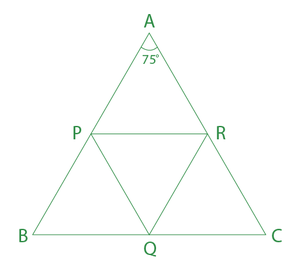∠PBQ = ∠QPB = x° ( since BQ = PQ)

∠RCQ = ∠QRC = y°  ( since QC=QR)

Now in ∆ABC,

∠ABC + ∠ACB + ∠BAC = 180°

=> x + y + 75° = 180°

=> x + y = 105°     ————-(1)

In ∆BPQ,

∠BPQ + ∠PBQ + ∠PQB = 180°

=> x + x + ∠PQB = 180°

=> ∠PQB = 180° – 2x   ———-(2)

In ∆CRQ,

∠QRC + ∠RCQ + ∠RQC = 180°

=> y + y + ∠RQC = 180°

=> ∠RQC = 180° – 2y   ———-(3)

Adding equation (2) and (3) ,we get

∠PQB + ∠RQC = 180° – 2x + 180° – 2y

= 360° – 2(x+y)

= 360° – 2×105°

= 150°

Here, ∠PQR ,∠PQB, ∠RQC lie in a straight line, so the sum of these angles be 180°.

∠PQR = 180° – (∠PQB + ∠RQC)

= 180° – 150°

∠PQR =  30°

∴ The value of ∠PQR = 30°

Que.10 A can finish a piece of work in 16 days and B can finish it in 12 days. They worked together for 4 days and then A left. B finished the remaining work. For how many days did B work to finish the work completely?

A) 6 days

B) 4 days

C) 7 days

D) 9 days

Explanation:

Total work( LCM of 16 and 12) = 48 units

A’s 1 day work = 48/16 = 3 units

B’s 1 day work = 48/12 = 4 units

(A+B)’s 4 days work = 4 × (3 + 4) = 28 units

Remaining work = 48 – 28 = 20 units

Remaining work done by B in = 20/4 = 5 days

∴ Total time taken by B to finish it completely =(4 + 5) days = 9 days.

Que.11 The value of (sin23°cos67°+sec52°sin38° + cos23°sin67°+cosec52°cos38°)/(cosec²20°- tan²70°)

A) 1

B) 2

C) 3

D) 0

Explanation :

(sin 23°cos 67° + sec 52°sin 38° + cos 23°sin 67° + cosec 52°cos 38°)/(cosec²20° – tan²70°)

= [sin 23°cos(90°-23°) + 1/cos 52° × sin(90°-52°) + cos 23°sin(90°-23°) + 1/sin 52° × cos(90°-52°)] /[cosec²(90°-70°) – tan²70°]

= (sin²23° + 1 + cos²23° + 1)/(sec²70° – tan²70°)

= (1+2)/1

= 3

Important points :

cos(90° – x) = sin x  ; sin(90°-x) = cos x

sin x = 1/cosec x   ;  cos x = 1/sec x

sin²x + cos²x = 1

sec²x – tan²x = 1

Que.12 LCM of two numbers is 56 times of their HCF, with the sum of their HCF and LCM being 1710. If one of the two numbers is 240, then what is the other number?

A) 210

B) 170

C) 57

D) 160

Explanation :

LCM = 56 × HCF

HCF + LCM = 1710

=> HCF + 56 HCF = 1710

=> HCF = 1710/57

=> HCF = 30

Now, LCM = 56 × 30 = 1680

Let, other number be P.

We know,

LCM × HCF = products of two numbers

1680 × 30 = 240 × P

=> P = (1680×30)/240

∴ P = 210

∴ The other number is = 210

Que.13 The following bar graph shows exports of cars of type A and B (in Rs. millions) from 2014 to 2018.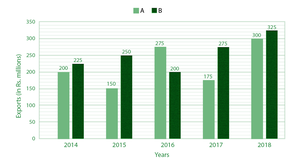What is the ratio of the total exports of cars of type A in 2014 and 2017 to the total exports of cars of type B in 2015 and 2016?

A) 5 : 6

B) 11 : 10

C) 6 : 7

D) 7 : 9

Explanation :

The total exports of cars of type A in 2014 and 2017 = ( 200 +175) = 375

The total exports of cars of type B in 2015 and 2016 = 250 + 200 = 450

Required ratio = 375 : 450 = 5 : 6

∴ The ratio of the total exports of cars of type A in 2014 and 2017 to the total exports of cars of type B in 2015 and 2016 is = 5 : 6

Que.14 A certain sum is deposited for 4 years at a rate of 10% per annum on compound interest compounded annually. The difference between the interest at the end of 2 years and that at the end of 4 years is Rs.5082. Find the sum.

A) 15,000

B) 18,000

C) 20,000

D) 25,000

Explanation :

Let the principal be 100x

Rate = 10%

Successive rate for 2 years

= 10 +10 + (10×10)/100  = 21%

Interest earned at the end of 2 years = 21% of 100x = 21x

Successive rate for 3 years

= 10 + 21 + (10×21)/100  = 33.1

Successive rate for 4 years

= 10 + 33.1 + (10×33.1)/100  = 46.41%

Interest earned at the end of 4 years = 46.41% of 100x = 46.41x.

Difference between the interest at the end of 2 years and that at the end of 4 years

= ( 46.41x – 21x)%  = 25.41% of x

According to question,

25.41% of x = 5082

100% of x = (5082/25.41) × 100 = 20,000

∴The required sum will be 20,000.

Que.15 An equilateral triangle ABC is inscribed in a circle with centre O. D is a point of the minor arc BC and ∠CBD=40°. Find the value of ∠BCD?

A) 30°

B) 20°

C) 35°

D) 45°

Explanation: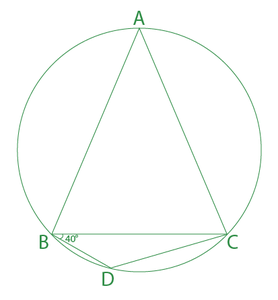∵ ∆ABC is an equilateral triangle, so all angles are equal.

∠ABC = ∠ACB = ∠BAC = 60°

Here, ABDC is a cyclic quadrilateral, so the sum of its opposite angles is 180°.

∠BAC + ∠BDC = 180°

=> ∠BDC = 180° – 60° = 120°

In ∆BDC ,

∠CBD + ∠BCD + ∠BDC = 180°

=> ∠BCD = 180° – ∠CBD – ∠BDC

=> ∠BCD = 180° – 40° – 120°

∴ ∠BCD = 20°

∴ The value of ∠BCD = 20°.

Que.16 The length of the three sides of a right-angled triangle are (x-1),(x+1) and (x+3) cm respectively. The hypotenuse of the right angled triangle is –

A) 6 cm

B) 8 cm

C) 10 cm

D) 15 cm

Explanation :

We know, in a right angled triangle hypotenuse is the largest side and the formula is

(Hypotenuse)² = (Base)² + (Height)²

=> (x + 3)² = (x -1)² + (x+1)²

=> x² + 6x + 9 = x² -2x +1 + x² + 2x + 1

=> x² – 6x – 7 = 0

=> x² – 7x + x – 7 = 0

=> (x – 7)(x + 1) = 0

=> x + 1 = 0 , x= -1 is not possible.

=> x – 7 = 0

=> x = 7

=>(x + 3) = 7+ 3 = 10 cm

∴ The hypotenuse of the right angled triangle is 10 cm.

Directions (Q17-18) The given histogram represents the marks of students in the Mathematics tests of a certain class. The total number of students is 350.

Study the graph and answer the question that follows.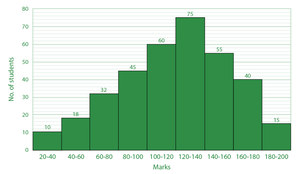Que.17 What is the ratio of the total number of students who scored 140 marks and above to the total number of students who scored marks between 60 to 120?

A) 9 : 11

B) 11 : 9

C) 137 : 110

D) 110 : 137

Explanation :

Total number of students scored 140 marks and above = (55 + 40 + 15) = 110

Total number of students scored marks between 60 to 120 =(32 + 45 + 60) = 137

Required ratio = 110 : 137

∴ The ratio of the total number of students who scored 140 marks and above to the total number of students who scored marks between 60 to 120 is

110 : 137.

Que.18 The ratio of monthly incomes of A and B is 11:13 and the ratio of their expenditure is 9:11. If both of them manage to save Rs.4000 per month, then find the difference in their incomes.

A) 2500

B) 3000

C) 4000

D) 4500

Explanation :

Let, monthly incomes of A and B are 11x and 13x respectively and expenditure be 9y and 11y .

Savings of A = 11x – 9y

Savings of B = 13x – 11y

Savings of A = Savings of B

11x – 9y = 13x – 11y

2y = 2x

x = y = 1 unit

According to question,

11x – 9y = 4000

11 units – 9 units = 4000

1 unit = 2000.

Difference of their incomes

= (13x – 11x)

= 2x

= 4000

∴ The difference of their incomes = Rs.4000

Que.19 A solid cube of side 8 cm dropped into a rectangular container of length 16 cm ,breadth 8 cm and height 15 cm which is partly filled with water. If the cube is completely submerged, then the rise of water level is –

A) 6 cm

B) 4 cm

C) 2.5 cm

D) 5.5 cm

Explanation :

The water level increased means its height increased.

Let, the water level increase = h cm

According to the question,

The volume of cube = volume of the water displaced (because the cube is completely submerged)

8³ = 16 × 8 × h

=> h = (8×8×8)/(16×8)

=> h = 4

∴ The water level increased by 4 cm.

Directions (Q20-21). The number of cars passing the road near a colony from 6 am to 12 noon has been shown in the following histogram.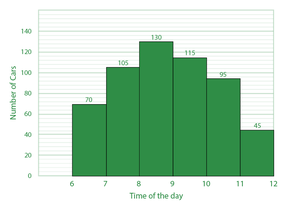Que.20 What is the ratio of the number of cars passed between 6 am and 8 am to the number of cars passed between 9 am to 11 am?

A) 3 : 4

B) 4 : 3

C) 6 : 5

D) 5 : 6

Explanation:

Total number of cars passed between 6 am to 8 am = (70 +105) = 175

Total number of cars passed between 9 am to 11 am = (115 + 95) = 210

Required ratio = 175 : 210 = 5 : 6.

∴ The ratio of the number of cars passed between 6 am and 8 am to the number of cars passed between 9 am to 11 am is 5: 6.

Que.21 An item costs Rs400 During a festival sale, a company offers a sale discount that offers x% off on its regular price along with a discount coupon of 10%. The price of the item after using both the sale discount and the discount coupon is Rs.216. What is the value of x?

A) 30

B) 35

C) 40

D) 50

Explanation :

Cost price (CP) = 400

Selling price (SP) = 216

Discount = (400-216) = 184

Discount % = (184/400) × 100 = 46%

According to question,

(-x)+(-10)+[(-x)×(-10)]/100 = -46% ( Here negative sign represents that their is a discount)

=> -x – 10 + x/10 = -46

=> -9x = -360

∴ x = 40

∴ The value of x = 40.

Que.22  If x + y + 3 = 0, then find the value of x³ + y³ – 9xy + 9.

A) – 18

B) 18

C) 27

D) – 12

Explanation :

x + y + 3 = 0

=> x + y = -3

=> (x + y)³ = (-3)³

=> x³ + y³ +3xy(x + y) = -27

=> x³ + y³ + 3xy(-3) = -27

=> x³ + y³ -9xy = -27

=> x³ + y³ – 9xy + 9 = -27 + 9 = -18

∴ The value of x³ + y³ – 9xy + 9 = -18

Que.23 AB is a diameter of a circle with a centre O. A tangent is drawn at point A. C is a point on the circle such that BC produced meets the tangent at P. If ∠APC = 62°, then find the measure of the minor arc AC.

A) 46°

B) 50°

C) 56°

D) 60°

Explanation :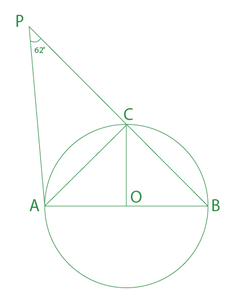Diameter AB is perpendicular to tangent AP. So,

∠BAP = 90°

∠ACB = 90°

∠ACP = 180° – ∠ACB

=> ∠ACP = 180° – 90° = 90°

Now from ∆APC,

∠PAC = 180° – ∠APC – ∠ACP = 180° – 62° – 90°

∠PAC = 28°

∠OAC = 90° – ∠PAC = 90° – 28°

∠OAC = 62°

∠OAC = ∠OCA =62°   (because OC=OA=radius)

Minor arc of AC =∠AOC

In ∆AOC,

∠AOC + ∠OCA + ∠OAC = 180°

=> ∠AOC = 180° – 62° – 62°

=> ∠AOC = 56°

∴ The value of minor arc AC = 56°.

Que.24 The monthly expenditure of a family on different heads is shown in the following pie chart.

Expenditure of different heads –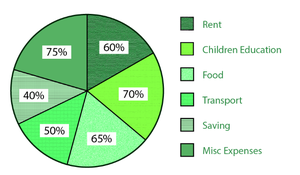The amount spends on children’s education, Transport and rent is what percentage of the total earnings?

A) 50%

B) 40%

C) 30%

D) 60%

Explanation :

Total expenditure = 360°

Total expenditure on children education, transport and rent = (70°+50°+60°) =180°

Required percentage =(180°/360°)×100% = 50%

∴The amount spend on children education, transport and rent is 50% percentage of the total earnings

Que.25 Find the value of the following expression-

372 ÷ 56 × 7 – 5 + 2

A) 38

B) 43.5

C) 47.20

D) 49.90

Explanation :

372 ÷ 56 × 7 – 5 + 2

= (372/56) × 7 – 5 + 2

= (93/14) × 7 – 5 + 2

= 93/2 – 3

= (93-6)/2

= 87/2

= 43.5

∴The required value= 43.5

My Personal Notes arrow_drop_up
Recommended Articles
Page :